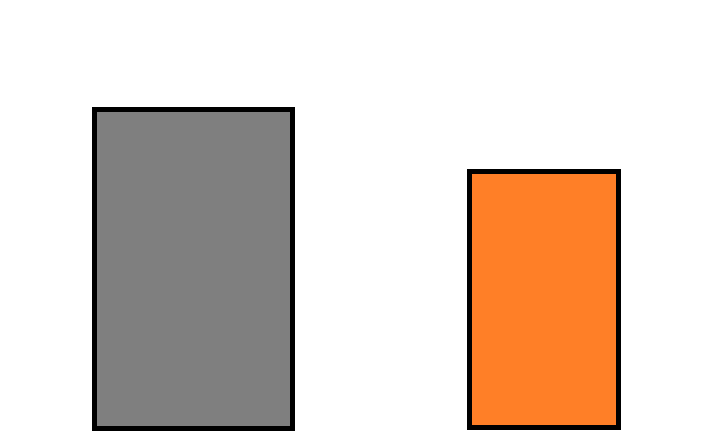﻿ How to Find the X and Y Coordinates of an Object in an Image in Python using OpenCV ﻿# How to Find the X and Y Coordinates of an Object in an Image in Python using OpenCVIn this article, we show how to find the x and y coordinates of an object in an image in Python using the OpenCV module.

OpenCV is very dynamic in which we can first find all the objects (or contours) in an image using the cv2.findContours() function.

We can then find the x and y coordinates of the contour using a little bit of custom code.

In our code, we'll find beginning and end x coordinates and the beginning and end y coordinates. We'll also show how to find the center x and y coordinates of each contour.

In this example, we will be working with the following image shown below.As you can see in the above image, there are 2 rectangles, which we will say represents boxes.

We will find the x and y coordinates of both of the figures in the image above.

Below we'll see how this works in code.

The code to find the x and y coordinates of both figures is shown below.

Let's now go over this code.

First, we import OpenCV using the line, import cv2

Next, we read in the image, which in this case is, Boxes.png

We create the variable, original_image, to store the original image that will undergo modification throughout the code.

We create a grayscale version of the image and then find the Canny edges, which helps simplify the image for cvs.findContours() function. The cv2.findContours() function finds all external contours in our image, which finds the unique objects in an image.

We then sort this list from largest to smallest using the sorted() function. We store this sorted list into the variable, sorted_contours. We sort a list from largest to smallest by the attribute, reverse=True. To sort a list from smallest to largest by the attribute, reverse= False, or by specifying nothing in the reverse attribute, as reverse=False, is implied if not specified.

Now that the list is sorted from largest to smallest, the first item in the list is the largest item, which represents the largest object in our image. We get the largest item with the code, largest_item= sorted_contours. The other object then is the smallest, smallest_item= sorted_contours.

We first find the x and y coordinates of the largest item.

We first compute the moments of the larger item, which will then allow us to compute the center x and y coordinates.

We then create a tuple of variables, x,y,w,h, and set it equal to cv2.boundingRect(). The x stands for the first x coordinate. The y stands for the first y coordinate. The w stands for the width of the object. The h stands for the height of the object.

Since we know the first x coordinate and the width, we can calculate the end x coordinate by adding the width to the x coordinate.

Since we know the first y coordinate and the height, we can calculate the end y coordinate by adding the height to the y coordinate.

We then do the same for the smaller object in the image.

Once we run this program, we get the following output.

We show the image using matplotlib with grids so you can directly compare the coordinates.

This is shown below.You can see the x and y coordinates output by the program accurately lines up with each of the objects (contours) of the image.

And this is how we can find the x and y coordinates of an object in an image in Python using OpenCV.

Related Resources